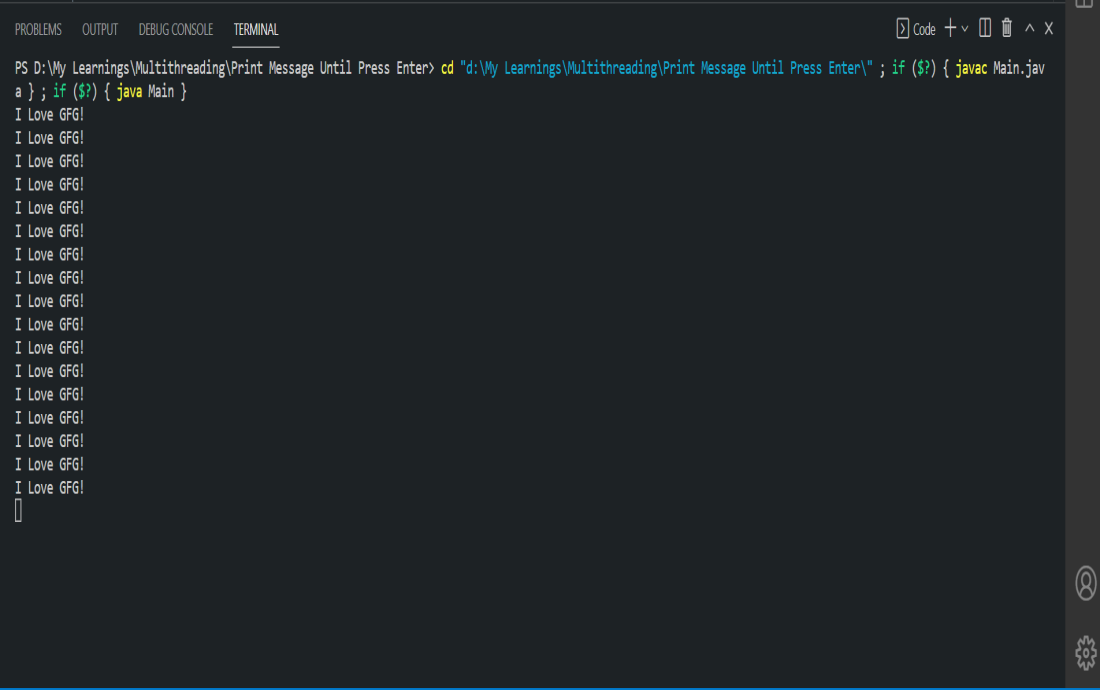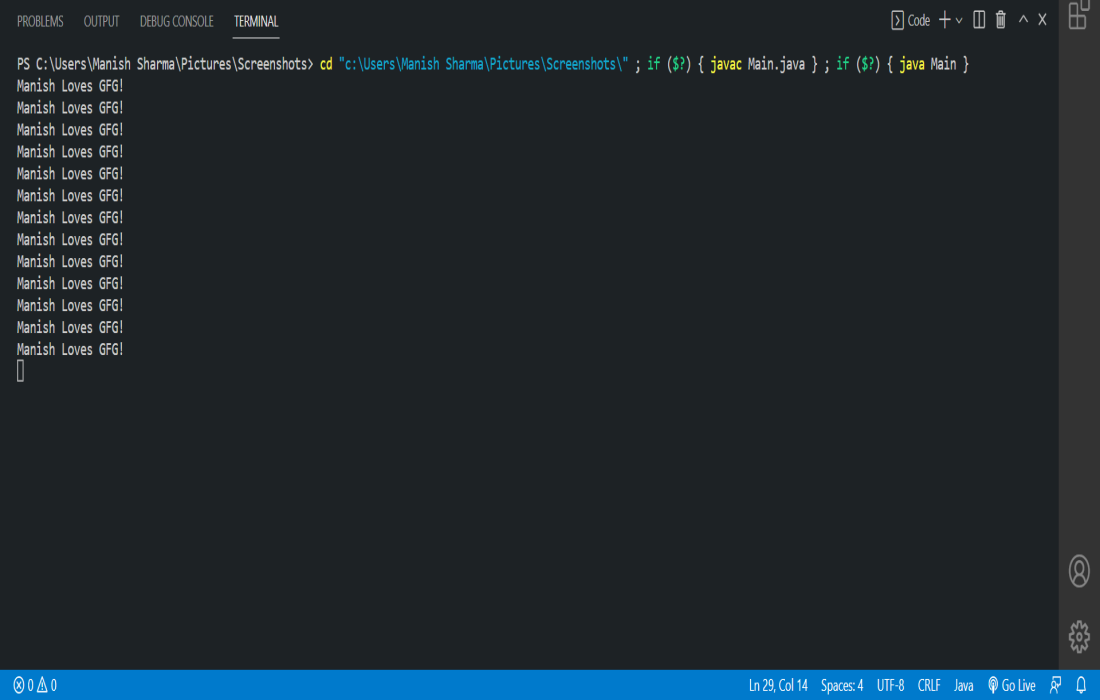Open in App
Not now

# Java Multithreading – One Thread to Take Input, Another to Print on Console

• Difficulty Level : Medium
• Last Updated : 07 Feb, 2023

Multithreading is a concept in which our program can do two or more tasks at the same time. Thread is the execution unit of any process. Every process has at least one thread which is called main thread. In this article, we will create a Java program that will do printing on the console until the user gives input. Here, we need two threads. One thread do printing and another waits for user input.

### Java Program and Explanation

Here, one thread is printing on the console and while the other thread will wait for user input. Main class is executed on the parent thread and also it creates a child thread that does printing on the console.

• Waits for user input.

• Do printing.

## Java

 `// fileName - GeeksForGeeks.java`   `import` `java.lang.*;`   `// needs to be inherited from` `// Thread class for multithreading` `public` `class` `GeeksForGeeks ``extends` `Thread {` `    ``private` `String mssg;`   `    ``// constructor` `    ``GeeksForGeeks(String mssg)` `    ``{` `        ``this``.mssg = mssg;` `    ``}`   `    ``// invoked when we` `    ``// call start() method` `    ``public` `void` `run()` `    ``{` `        ``// prints only when start variable` `        ``// is true (variable present in Main class)` `        ``while` `(Main.start == ``true``) {` `            ``System.out.println(``this``.mssg);` `            ``try` `{` `                ``// put thread on waiting` `                ``// state for 1500ms` `                ``Thread.sleep(``1500``);` `            ``}` `            ``catch` `(Exception err) {` `            ``}` `        ``}` `    ``}` `}`

Explanation of the above code:

• We extend our class to Thread class to make our program multithreaded.
• its run() method is invoked internally whenever we invoke the start() method.
• run() methods do printing until the start variable value is true.

## Java

 `// fileName - Main.java`   `import` `java.lang.*;` `import` `java.util.Scanner;`   `public` `class` `Main {` `    ``// public static variable and it's` `    ``// value will be false when user enter input` `    ``public` `static` `boolean` `start = ``true``;` `    ``public` `static` `void` `main(String args[])` `    ``{` `        ``GeeksForGeeks newThread = ``new` `GeeksForGeeks(``"I Love GFG!"``);`   `        ``// child thread execution` `        ``// starts here` `        ``newThread.start();`   `        ``Scanner sc = ``new` `Scanner(System.in);`   `        ``// parent thread waiting for user input here` `        ``// parent thread is at waiting state at this line` `        ``sc.nextLine();`   `        ``// when user gave any input then the value` `        ``// of this variable changed to be false` `        ``// it will tells the child thread that` `        ``// user gave input and now stop printing` `        ``Main.start = ``false``;` `    ``}` `}`

Explanation of the above code:

• It creates an object of GeeksForGeeks Class which extends the Thread class.
• After this, it invokes the start() method which executes all the code of the GeeksForGeeks run() method on a different thread.
• After that, It waits for user input.

### Output: printing and waiting for user input at the same timeprinting and waiting for user input at the same time

## Java

 `/*package whatever // do not write package name here */`   `// This program may not work on at gfg IDE` `// run it on your local system` `import` `java.io.*;` `import` `java.lang.*;` `import` `java.util.Scanner;`   `// needs to be inherited from Thread` `// class for multithreading` `class` `GeeksForGeeks ``extends` `Thread {` `    ``private` `String mssg;`   `    ``GeeksForGeeks(String mssg)` `    ``{` `        ``this``.mssg = mssg;` `    ``}`   `    ``// invoked when we` `    ``// call start() method` `    ``public` `void` `run()` `    ``{` `        ``// prints only when start variable` `        ``// is true (variable present in Main class)` `        ``while` `(Main.start == ``true``) {` `            ``System.out.println(``this``.mssg);` `            ``try` `{` `                ``// put thread on waiting` `                ``// state for 1000ms` `                ``Thread.sleep(``1000``);` `            ``}` `            ``catch` `(Exception err) {` `            ``}` `        ``}` `    ``}` `}`   `public` `class` `Main {` `    ``// public static variable and it's value` `    ``// will be false when user enter input` `    ``public` `static` `boolean` `start = ``true``;` `    ``public` `static` `void` `main(String[] args)` `    ``{` `        ``GeeksForGeeks newThread = ``new` `GeeksForGeeks(``"Manish Loves GFG!"``);`   `        ``// child thread execution` `        ``// starts here` `        ``newThread.start();`   `        ``Scanner sc = ``new` `Scanner(System.in);`   `        ``// parent thread waiting for user input here` `        ``// parent thread is at waiting state at this line` `        ``sc.nextLine();`   `        ``// when user gave any input then the value` `        ``// of this variable changed to be false` `        ``// it will tells the child thread that` `        ``// user gave input and now stop printing` `        ``Main.start = ``false``;` `    ``}` `}`

### Output: printing and waiting for user input at the same timeprinting and waiting for user input at the same time

My Personal Notes arrow_drop_up
Related Articles